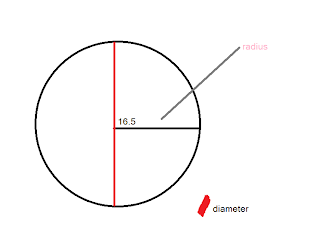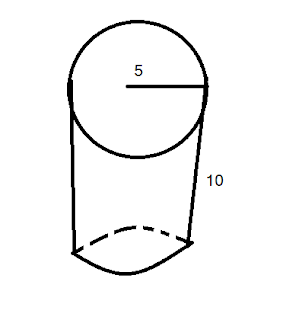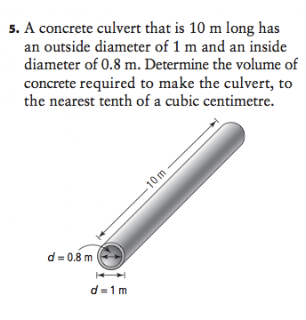## Wednesday, March 23, 2011

### Jocelyn Leclaire Surface Area Post

Today in class we did a few questions, and we got taught some new formulas.

Some of the questions that we did was:So Mr.Harbeck gave us a circle that has a radius of 16.5 cm.
The next step for us to do was to find the diameter.
The formula to find the diameter is:If the image is hard to see or it doesn't work it is:
d=2r
d=2x16.5
d= 33 cm

So the next thing we had to find out the circumfrence:
If you don't know what circumfrence is, it is this:The formula to find the circumfrance for this question is:
C=πd
C=3.14x33=
C=103.6The next thing we had to do is find the area of this circle:The formula to find area is
A=πxrxr
A=3.14x16.5x16.5
A= 854.87

Once I demonstrated that to you, I will not post any more pictures.
Another circle we did is one which has a diameter of 15.
For us to find the Radius of a diameter the formula is
R= D/2
R= 15/2
R=7.5

After we find the Radius we should find the circumfrance(we already have the diameter)
C=πd
C=3.14x15
C=47.1

After the circumfrence the last thing we have to do is to find the area.
A=πxrxr
A=3.14x7.5x7.5
A=176.35

Another thing we did in class today was we used a cylinder such as this.After we break it down it should be like thisSince we have the radius we should go onto the diameter
D=2r
D=2x5
D=10 cm

After the diameter, we should go onto the circumfrence:
C= πD
C=3.14x10
C=31.4

LxW=rectangle
31.4x10
=314 cm2

After circumfrence we should go to Area.

A=πxrxr
A=3.14x5x5=
A= 78.5

TSA=
471 Cm2

Another formula we did to make everything shorter that I put up there was
(2πxrxr)+2xrcrπxh)=
(2x3.14x5x5)+2x5x3.14x10)=

So one of the questions that I'm going to be doing is:Since it gives us the diameter, I started off with the radius.
R=D/2
R=7/2
R=3.5

Then I went to the Circumfrence:
C=πD
C=3.14x7
C=21.98

LxW= 7x2198
= 153.86

After that, I went to the area:
A=πxrxr
A=3.14x3.5x3.5
A=213.445Another question is:D=R2
D=3x2
D=6

C=πD
C=3.14x6
C=18.84

LxW
5x18.84=94.2

Next we do the Area
A=πxrxr
A=3.14x3x3
A=38.26

28.26+ 18.84
----------------
47.1
+ 94.2
------------
141.3

Another Question is:D= Rx2
D=3x2
D=6

C=πD
C=3.14x6
C=18.84

LxW
5x18.84=
94.2

A=πxrxr
A-3.14x3x3
A=28.26

28.26
+ 18.84
--------------
47.1
+94.2
-------------------
141.3 cm2